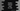# Python program to remove one occurrence of a word in a list## Python program to remove the kth occurrence of a word in list :

In this tutorial, we will learn how to remove the kth occurrence of a word in a given list. The list is given. It contains words and most words are repeated. We will create one function to remove the kth occurrence of one word if it is available in the list. If it is not available, it will show one message.

## Python program :

The python program is as like below :

``````# 1
def removeWord(list, word, k):
n = 0
#2
for i in range(0, len(list)):
if(list[i] == word):
n += 1
#3
if(n == k):
del(list[i])
return True

return False

#4
list = ['hello', 'world', 'hello', 'world', 'hello', 'world']

#5
if(removeWord(list, input("Enter a word : "), int(input("Enter k : ")))):
print("The list is updated : ", list)
else:

### Explanation :

The commented numbers in the above program denote the step numbers below :

1. removeWord function is used to remove the kth occurrence of a word in a given list. It takes three arguments : the list, word to remove and the value of k.
2. n variable is initialized to 0. This variable will hold the current count of the word. Using one for loop, we are iterating through the list of words one by one. If the current value is equal to the given word, increase the value of n by 1.
3. Check if the value of n is equal to k or not. If yes, delete the element of index i and return True. Else, return False once the loop ends.
4. list is the given list of words.
5. We are taking the word and k as input from the user. If the word is found, it prints the updated list. Else, it prints one message that the word is not found.

### Sample Output :

``````Enter a word : hfdal
Enter k : 2